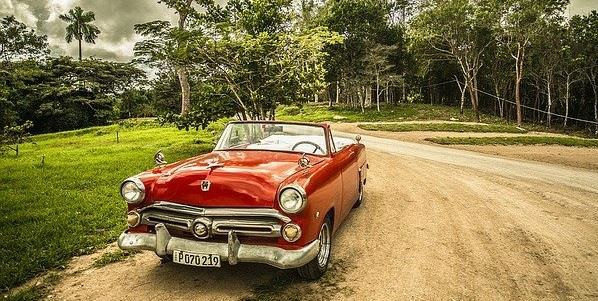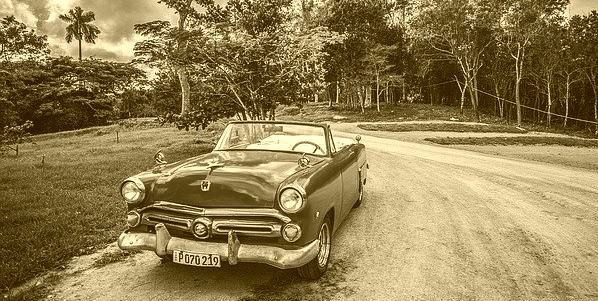# How to convert a colored image to Sepia image using Java OpenCV library?

## Algorithm to convert a colored image to sepia −

• Get the red green blue values of each pixel

• Get the average of these 3 colors.

• Define the depth and intensity values (ideally 20, and 30).

• Modify the values as −

• red = red + (depth*2).

• Green = green +depth.

• blue = blue-intensity.

• Make sure that the modified values are between 0 to 255.

• Create a new pixel value from the modified colors and set the new value to the pixel.

## Implementation in Java

• Get the height and width of the image.

• Using nested for loops traverse through each pixel in the image.

• Get the pixel value using the getRGB() method.

• Create a Color object by passing the above-retrieved pixel value as parameter.

• Get the red, green, blue values from the color object using the getRed(), getGreen() and getBlue() methods respectively.

• Calculate the new red, green and blue values as specified in the algorithm.

• Create a new pixel with the modified RGB values.

• Set the new pixel value(s) using the setRGB() method.

## Example

import java.io.File;
import java.io.IOException;
import java.awt.Color;
import java.awt.image.BufferedImage;
import javax.imageio.ImageIO;
public class Color2Sepia {
public static void main(String args[])throws IOException {
File file= new File("D:\Images\cuba.jpg");
for (int y = 0; y < img.getHeight(); y++) {
for (int x = 0; x < img.getWidth(); x++) {
//Retrieving the values of a pixel
int pixel = img.getRGB(x,y);
//Creating a Color object from pixel value
Color color = new Color(pixel, true);
//Retrieving the R G B values
int red = color.getRed();
int green = color.getGreen();
int blue = color.getBlue();
int avg = (red+green+blue)/3;
int depth = 20;
int intensity = 30;
red= avg+(depth*2);
green = avg+depth;
blue = avg-intensity;
//Making sure that RGB values lies between 0-255
if (red > 255)red = 255;
if (green > 255)green = 255;
if (blue > 255)blue = 255;
if (blue<0)blue=0;
//Creating new Color object
color = new Color(red, green, blue);
//Setting new Color object to the image
img.setRGB(x, y, color.getRGB());
}
}
//Saving the modified image
file = new File("D:\Images\sepia_image2.jpg");
ImageIO.write(img, "jpg", file);
System.out.println("Done...");
}
}

## Input## Output